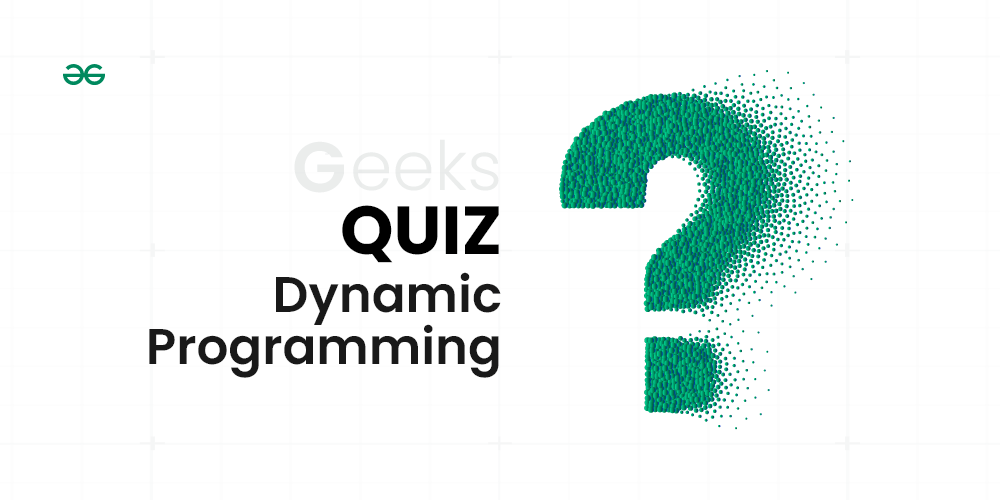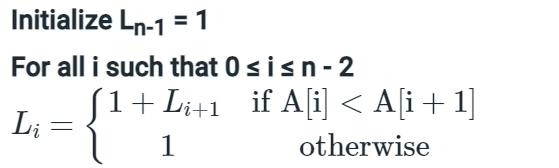# Top MCQs on Dynamic Programming with Answers

Dynamic Programming (DP) is defined as a technique that solves some particular type of problems in Polynomial Time. Dynamic Programming solutions are faster than the exponential brute method and can be easily proved their correctness.
More on Dynamic ProgrammingDynamic Programming Quiz

 Question 1

Which of the following standard algorithms is not Dynamic Programming based?Bellman–Ford Algorithm for single source shortest pathFloyd Warshall Algorithm for all pairs shortest paths0-1 Knapsack problemPrim's Minimum Spanning Tree

Question 1-Explanation:

Prim's Minimum Spanning Tree is a Greedy Algorithm. All others are dynamic programming based.

Hence (D) is the correct answer.

 Question 2

We use dynamic programming approach whenWe need an optimal solutionThe solution has optimal substructureThe given problem can be reduced to the 3-SAT problemIt's faster than Greedy

Question 2-Explanation:

We use dynamic programming approach when the solution has an optimal substructure.

Hence Option(B) is the correct answer.

 Question 3

An algorithm to find the length of the longest monotonically increasing sequence of numbers in an array A[0 :n-1] is given below. Let Li denote the length of the longest monotonically increasing sequence starting at index i in the array.Equation

Which of the following statements is TRUE?The algorithm uses dynamic programming paradigmThe algorithm has a linear complexity and uses branch and bound paradigmThe algorithm has a non-linear polynomial complexity and uses branch and bound paradigmThe algorithm uses divide and conquer paradigm.

Question 3-Explanation:

The algorithm uses a dynamic programming paradigm. Dynamic programming is a technique for solving problems by breaking them down into smaller subproblems and using the solutions to the subproblems to solve the original problem. In this case, the algorithm breaks down the problem of finding the longest monotonically increasing sequence into subproblems of finding the longest monotonically increasing sequence starting at each index in the array. The algorithm then uses the solutions to these subproblems to find the solution to the original problem.

Here is the mathematical explanation:

Let L[i] denote the length of the longest monotonically increasing sequence starting at index i in the array A[0:n - 1]. Then, the algorithm can be written as follows:

L[n - 1] = 1

for i in range(n - 2, -1, -1):

L[i] = 1 if A[i] >= A[i + 1] else 1 + L[i + 1]

Hence Option(A) is correct.

 Question 4

Which of the following is NOT a characteristic of dynamic programming?Memoization, which involves storing the results of expensive function calls and reusing them.Breaking a problem into smaller overlapping subproblems.Solving problems in a sequential manner.Dynamic programming can be used for problems where the solution has an optimal substructure.

Question 4-Explanation:

Option (A): In dynamic programming Break down the given problem in order to begin solving it. If you see that the problem has already been solved, return the saved answer. If it hasn’t been solved, solve it and save it. This is usually easy to think of and very intuitive, This is referred to as Memoization.

Option (B): It is a big hint for DP if the given problem can be broken up into smaller sub-problems, and these smaller subproblems can be divided into still smaller ones, and in this process, you see some overlapping subproblems.

Option(C): Dynamic problems can never be solved in a sequential manner.

Option(D): Dynamic programming can be used for problems where the solution has an optimal substructure. this statement is also correct.

Hence (C) is the correct answer.

 Question 5

Four matrices M1, M2, M3 and M4 of dimensions pxq, qxr, rxs and sxt respectively can be multiplied is several ways with different number of total scalar multiplications. For example, when multiplied as ((M1 X M2) X (M3 X M4)), the total number of multiplications is pqr + rst + prt. When multiplied as (((M1 X M2) X M3) X M4), the total number of scalar multiplications is pqr + prs + pst. If p = 10, q = 100, r = 20, s = 5 and t = 80, then the number of scalar multiplications needed is248000440001900025000

Question 5-Explanation:

It is basically matrix chain multiplication problem. We get minimum number of multiplications using ((M1 X (M2 X M3)) X M4). Total number of multiplications = 100x20x5 (for M2 x M3) + 10x100x5 + 10x5x80 = 19000.

Hence (C) is the correct answer.

 Question 6

The subset-sum problem is defined as follows. Given a set of n positive integers, S = {a1 ,a2 ,a3 ,…,an} and positive integer W, is there a subset of S whose elements sum to W? A dynamic program for solving this problem uses a 2-dimensional Boolean array X, with n rows and W+1 columns. X[i, j],1 <= i <= n, 0 <= j <= W, is TRUE if and only if there is a subset of {a1 ,a2 ,...,ai} whose elements sum to j. Which of the following is valid for 2 <= i <= n and ai <= j <= W?X[i, j] = X[i - 1, j] ∨ X[i, j -ai]X[i, j] = X[i - 1, j] ∨ X[i - 1, j - ai]X[i, j] = X[i - 1, j] ∧ X[i, j - ai]X[i, j] = X[i - 1, j] ∧ X[i -1, j - ai]

Question 6-Explanation:

X[I, j] (2 <= i <= n and ai <= j <= W), is true if any of the following is true 1) Sum of weights excluding ai is equal to j, i.e., if X[i-1, j] is true. 2) Sum of weights including ai is equal to j, i.e., if X[i-1, j-ai] is true so that we get (j – ai) + ai as j See http://www.geeksforgeeks.org/dynamic-programming-subset-sum-problem/ for details.

Hence (B) is the correct answer.

 Question 7

In the above question, which entry of the array X, if TRUE, implies that there is a subset whose elements sum to W?X[1, W]X[n ,0]X[n, W]X[n -1, n]

Question 7-Explanation:

If we get the entry X[n, W] as true then there is a subset of {a1, a2, .. an} that has sum as W.

Hence (C) is the correct answer.

 Question 8

A sub-sequence of a given sequence is just the given sequence with some elements (possibly none or all) left out. We are given two sequences X[m] and Y[n] of lengths m and n respectively, with indexes of X and Y starting from 0. We wish to find the length of the longest common sub-sequence(LCS) of X[m] and Y[n] as l(m,n), where an incomplete recursive definition for the function l(i,j) to compute the length of The LCS of X[m] and Y[n] is given below:

```l(i,j) = 0, if either i=0 or j=0
= expr1, if i,j > 0 and X[i-1] = Y[j-1]
= expr2, if i,j > 0 and X[i-1] != Y[j-1] ```expr1 ≡ l(i-1, j) + 1expr1 ≡ l(i, j-1)expr2 ≡ max(l(i-1, j), l(i, j-1))expr2 ≡ max(l(i-1,j-1),l(i,j))

Question 8-Explanation:

In Longest common subsequence problem, there are two cases for X[0..i] and Y[0..j]

```1) The last characters of two strings match.
The length of lcs is length of lcs of X[0..i-1] and Y[0..j-1]
2) The last characters don't match.
The length of lcs is max of following two lcs values
a) LCS of X[0..i-1] and Y[0..j]
b) LCS of X[0..i] and Y[0..j-1]```

Hence (C) is the correct answer.

 Question 9

Consider two strings A = "qpqrr" and B = "pqprqrp". Let x be the length of the longest common subsequence (not necessarily contiguous) between A and B and let y be the number of such longest common subsequences between A and B. Then x + 10y = ___.33234334

Question 9-Explanation:

The LCS is of length 4. There are 3 LCS of length 4 "qprr", "pqrr" and qpqr   A subsequence is a sequence that can be derived from another sequence by selecting zero or more elements from it, without changing the order of the remaining elements. Subsequence need not be contiguous. Since the length of given strings A = “qpqrr” and B = “pqprqrp” are very small, we don’t need to build a 5x7 matrix and solve it using dynamic programming. Rather we can solve it manually just by brute force. We will first check whether there exist a subsequence  of length 5 since min_length(A,B) = 5. Since there is no subsequence , we will now check for length 4. “qprr”, “pqrr” and “qpqr” are common in both strings. X = 4 and Y = 3 .

X + 10Y = 34

Hence (D) is the correct naswer.

 Question 10

Let A1, A2, A3, and A4 be four matrices of dimensions 10 x 5, 5 x 20, 20 x 10, and 10 x 5, respectively. The minimum number of scalar multiplications required to find the product A1A2A3A4 using the basic matrix multiplication method is15002000500100

Question 10-Explanation:

We have many ways to do matrix chain multiplication because matrix multiplication is associative. In other words, no matter how we parenthesize the product, the result of the matrix chain multiplication obtained will remain the same. Here we have four matrices A1, A2, A3, and A4, we would have: ((A1A2)A3)A4 = ((A1(A2A3))A4) = (A1A2)(A3A4) = A1((A2A3)A4) = A1(A2(A3A4)). However, the order in which we parenthesize the product affects the number of simple arithmetic operations needed to compute the product, or the efficiency. Here, A1 is a 10 × 5 matrix, A2 is a 5 x 20 matrix, and A3 is a 20 x 10 matrix, and A4 is 10 x 5. If we multiply two matrices A and B of order l x m and m x n respectively,then the number of scalar multiplications in the multiplication of A and B will be lxmxn. Then, The number of scalar multiplications required in the following sequence of matrices will be : A1((A2A3)A4) = (5 x 20 x 10) + (5 x 10 x 5) + (10 x 5 x 5) = 1000 + 250 + 250 = 1500. All other parenthesized options will require number of multiplications more than 1500.

There are 30 questions to complete.

• Last Updated : 27 Sep, 2023